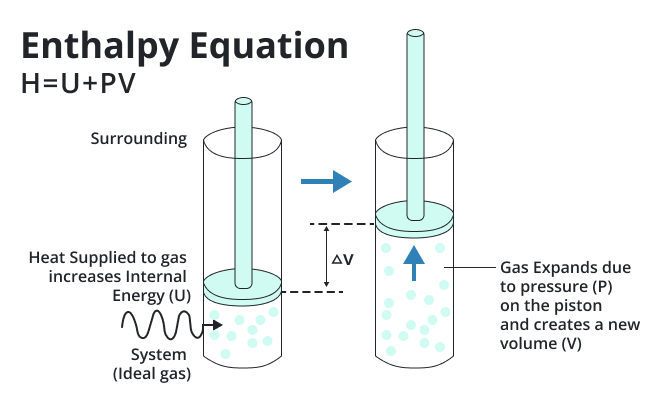# Enthalpy Calculator

Put the values and hit the calculate button to get the value of enthalpy using this enthalpy calculator.

Formula:
H = U + PV

Give Us Feedback

## Enthalpy Calculator

Enthalpy Calculator is a tool that is used to calculate the enthalpy of a substance or a chemical reaction.

## What is enthalpy?

Enthalpy is a thermodynamic parameter that is frequently employed in the context of chemical reactions and phase shifts to define the overall energy content of a system. It is essential for comprehending the energy transfers that take place during various processes.Enthalpy abbreviated as H is defined as the total of a system's internal energy U and the sum of the system's pressure P and volume V:

`H = U + PV`

Where

• H is the system's enthalpy, which is expressed in joules according to the International System of Units (SI).
• U is the system's internal energy, or the sum of all the energies held by the particles atoms, molecules, etc. that make up the system. Because of the interactions between the particles, it also contains kinetic energy and potential energy.
• P is the system's pressure, which is the force applied to the system's borders per unit of area measured in pascals in SI.
• V or the system's volume indicates how much room the system takes up.

Particular importance is given to enthalpy in chemical reactions. The change in enthalpy H that occurs during a chemical reaction at a constant pressure is referred to as the reaction's heat.

The amount of heat energy absorbed or emitted during the reaction is indicated by this. When H is negative, the reaction is exothermic, which means that heat is released into the environment. The reaction is endothermic, which means it takes in heat from the environment if H is positive.

## How to calculate enthalpy?

Example 1:

Determine the enthalpy using the data given

U = 21, P = 32, V = 45, H =?

Solution

Step 1:

Formula:

`H = U + PV`

Step 2:

Now we have to put values in the above formula:

H = 21 + (32)(45)

H = 21 + 1440

`H = 1461 joules`

Example 2:

Determine the enthalpy using the data given

U = 23, P = 43, V = 11, H =?

Solution

Step 1:

Formula:

`H = U + PV`

Step 2:

Now we have to put values in the above formula:

H = 23 + (43)(11)

H = 23 + 473

`H = 496 joules`

### Math Tools